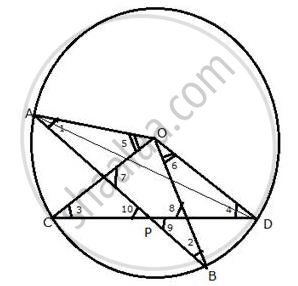Share

# Two Chords Ab and Cd Intersect at P Inside the Circle. Prove that the Sum of the Angles Subtended by the Arcs Ac and Bd at the Centre O is Equal to Twice the Angle Apc. - Mathematics

Course

#### Question

Two chords AB and CD intersect at P inside the circle. Prove that the sum of the angles
subtended by the arcs AC and BD at the centre O is equal to twice the angle APC.

#### SolutionGiven : two chords AB and CD intersect each other at P inside the circle. OA,
OB, OC and OD are joined.
To prove: ∠AOC + ∠BOD = 2∠APC
Proof: Arc AC subtends ∠AOCat the centre and ∠ADCat the remaining
Part of the circle.

Similarly,
From (3) and (4),
∠AOC + ∠BOD = 2∠APC

Is there an error in this question or solution?